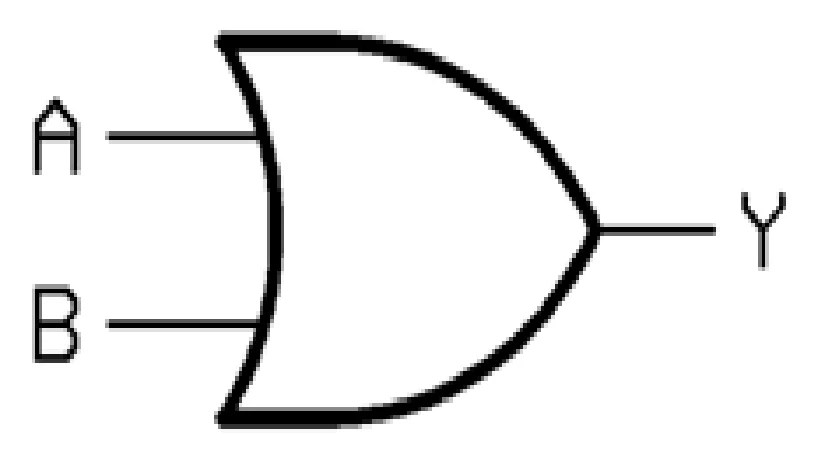# How to make an OR Gate using Diodes on Breadboard

I am sure you must be familiar with an OR Gate, it’s Truth Table, Logic symbol, and its working. But do you know, you can build your own OR Gate on Breadboard?  So in this post, you will learn how to make an OR Gate using Diodes on Breadboard.

**Read Similar Article: And Gate using Diodes

What is an OR Gate?OR gate is a Logic Gate and called so because OR means “to add”. There are two inputs and one output in an OR Gate.  So only when both inputs are zero, the output is zero and one in all the other cases.

You can also watch the video below for quick reference:

Truth Table:Components required:

1. 1N4007 Diode X 2

2. 1k Resistor

3. LED

4. 9 Volt Battery

5. Connecting Wires

SCHEMATIC:

This is the circuit we are going to build on Breadboard.

1. N terminal of diodes is connected together.

2. Logic inputs are given to P terminal of diodes.

3. End terminals of the Resistance are connected to N- terminals of the diode and LED respectively.

4. Output indicator i.e LED is connected from Resistor to Ground.

5. Ground- Negative terminal of the battery.

WORKING:

NOTE: When P-terminal of a Diode is at a higher potential (>.7v) then its n terminal, it acts like a short circuit. And when n terminal is at a higher potential, Diode act as an open circuit.

**from circuit diagram

CASE 1: Input A=0, Input B= 0

In this case, p-terminal of both diodes is at 0 volts w.r.t n-terminal which is  <.7v and hence acts like an open circuit. Thus no current flow from 1k resistor, then LED to Ground. Hence there is no voltage across LED and it remains off i.e, 0 at the output.

CASE 2: Input A=0, Input B= 1

In this case, p-terminal of diode A is at 0 volts w.r.t n-terminal which is  <.7v and hence acts like an open circuit. But p-terminal of diode B is at a higher potential than its n-terminal which is >.7v. Thus all current flow from 1k resistor, then LED to Ground. Hence there is some voltage across LED and it turns on i.e, 1 at the output.

CASE 3: Input A=1, Input B= 0

In this case, p-terminal of diode B is at 0 volts w.r.t n-terminal which is  <.7v and hence acts like an open circuit. But p-terminal of diode A is at a higher potential than its n-terminal which is >.7v. Thus all current flow from 1k resistor, then LED to Ground. Hence there is some voltage across LED and it turns on i.e, 1 at the output.

CASE 4: Input A=1, Input B= 1

In this case, p-terminal of both diodes is at higher potential w.r.t n-terminal which is  >.7v and hence acts like short circuits. Thus all current flow from 1k resistor, then LED to Ground. Hence there is some voltage across LED and it turns on i.e, 1 at the output.

** Hence we get 0(LED is off) at the output only when both the inputs are 0 as given in Truth Table.

Simulation:

Note: Before building the circuit on a breadboard, it is first simulated on “Every circuit” app. You can download the app from here.

** The circuit is exactly similar to what is given above.

** Slideshow

CASE 1: Input A=0, Input B= 0 , Output=0 (LED is OFF)

CASE 2: Input A=0, Input B= 1, Output=1 (LED is ON)

CASE 3: Input A=1, Input B= 0, Output=1 (LED is ON)

CASE 4: Input A=1, Input B= 1, Output=1 (LED is ON)

This slideshow requires JavaScript.

Let’s Make it!

1. Place the two diodes as shown below. N-terminal is the silver portion, P-terminal is the Black portion.

**Slideshow

This slideshow requires JavaScript.

2. Connect  N terminal of the Diodes together using wire as shown below:

**Slideshow

This slideshow requires JavaScript.

3. Connect two jumper wires, each at respective p-terminal of the diodes indicating input Logic.

This slideshow requires JavaScript.

4. Connect Battery to the breadboard as shown.5. Connect the resistor from n terminal of Diodes to Ground through LED.

**Slideshow

This slideshow requires JavaScript.

6. Now connect LED from resistor to Gnd(battery’s -ve terminal) .

**SlideshowCASE 1: Input A=0, Input B= 0 , Output=0 (LED is OFF)In this case, p-terminal of both diodes is at 0 volts w.r.t n-terminal which is  <.7v and hence acts like an open circuit. Thus no current flow from 1k resistor, then LED to Ground. Hence there is no voltage across LED and it remains off i.e, 0 at the output.

CASE 2: Input A=0, Input B= 1, Output=1 (LED is ON)In this case, p-terminal of diode A is at 0 volts w.r.t n-terminal which is  <.7v and hence acts like an open circuit. But p-terminal of diode B is at a higher potential than its n-terminal which is >.7v. Thus all current flow from 1k resistor, then LED to Ground. Hence there is some voltage across LED and it turns on i.e, 1 at the output.

CASE 3: Input A=1, Input B= 0, Output=1 (LED is ON)In this case, p-terminal of diode B is at 0 volts w.r.t n-terminal which is  <.7v and hence acts like an open circuit. But p-terminal of diode A is at a higher potential than its n-terminal which is >.7v. Thus all current flow from 1k resistor, then LED to Ground. Hence there is some voltage across LED and it turns on i.e, 1 at the output.

CASE 4: Input A=1, Input B= 1, Output=1 (LED is ON)In this case, p-terminal of both diodes is at higher potential w.r.t n-terminal which is  >.7v and hence acts like short circuits. Thus all current flow from 1k resistor, then LED to Ground. Hence there is some voltage across LED and it turns on i.e, 1 at the output.

** Hence we get 0(LED is off) at the output only when both the inputs are 0 as given in Truth Table.

You can also watch the video below for quick reference: